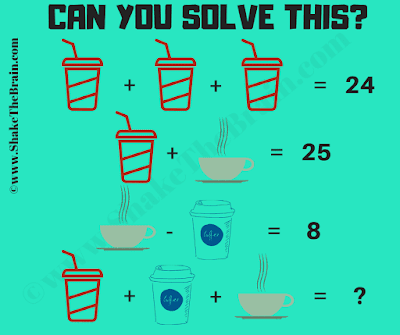This Math Picture Puzzle is a very easy math brain teaser. This can be easily solved by a school going, kid. In this math picture puzzle, you are shown some algebraic math equations. Solve these simultaneous math equations and find the value of the missing number which replaces question mark.Can you solve this Math Picture Puzzle?
Answer of this "Math Picture Puzzle for Kids", can be viewed by clicking on the answer button.